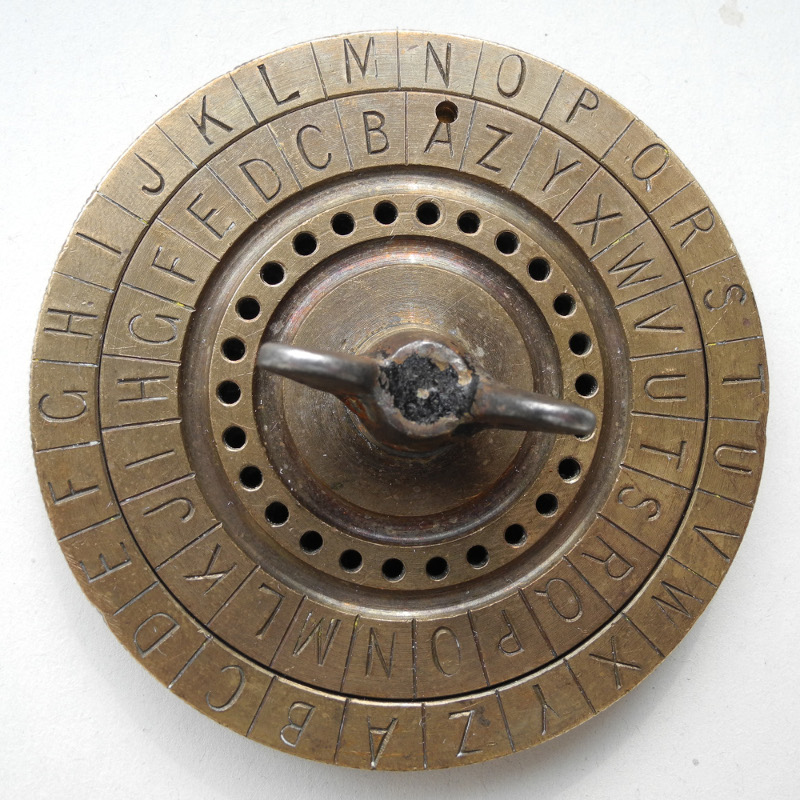# Solution to TUCTF 2017 The Neverending Crypto##### name:

The Neverending Crypto

crypto

50

#### Writeup

We where presented with a server that encrypted strings with a substitution cipher.

You where also able to give up to twenty characters that the server gave the solution for.

The server didn’t use the complete byte range and it was a hassle to find out what characters that was included in the substitution. The server leaked the plain texts from time to time, and the number of plain text strings that was encrypted was small, only nine different ones.

This meant that we could write some simple heuristics to decide which of the strings to send back, the same plain text character always encrypts to the same encrypted character, so we could look at what characters in the ecrypted text are equal to determine what plain text to send back.

Implemented like this:

``````import socket
import re
import string

sobj = socket.socket(socket.AF_INET,socket.SOCK_STREAM)
sobj.connect(("neverending.tuctf.com",12345))
sobj.recv(1024)

re_question = re.compile(r"is (?P<question>.*) decrypted")
re_match = re.compile(r"a encrypted is (?P<chr>.)")

def workit():
sobj.send("a\n")

hint =  sobj.recv(1024)
print "hint", repr(hint)
c = re_match.match(hint).groupdict()["chr"]

# What is :BB7RJBE>^R@BE8 decrypted?
question = re_question.findall(hint)
print "Question is", question
if question == question:
answer = "how many more??"
if question == question:
answer = "something here."
if question == question:
answer = "you got lucky.."
if question == question:
answer = "you have skills"
if question == question:
answer = "good work, more"
if question == question:
answer = "you crypto wiz!"
if question == question and question == question:
answer = "how many more??"
if question == question:
answer = "welcome, hacker"
if question == question and question == question and question == question:
answer = "dont forget to "
if answer == "":
print "I DO NOT KNOW"
import sys
sys.exit()